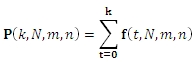# SQL Server HYPGEOM.INV function

HYPGEOMDISTPINV

Updated: 31 July 2010

Use HYPGEOMDISTPINV to calculate the inverse of the cumulative distribution function of the hypergeometric distribution.

The formula for the cumulative distribution function is:Syntax
SELECT [wctStatistics].[wct].[HYPGEOMDISTPINV] (
<@P, float,>
,<@Number_sample, float,>
,<@Population_s, float,>
,<@Number_population, float,>)
Arguments
@P
is the cumulative distribution function. @P is an expression of type float or of a type that can be implicitly converted to float
@Number_sample
is the size of the sample. @Number_sample  is an expression of type float or of a type that can be implicitly converted to float
@Population_s
is the number of successes in the population. @Population_s is an expression of type float or of a type that can be implicitly converted to float
@Number_population
is the population size. @Number_Population is an expression of type float or of a type that can be implicitly converted to float
Return Types
float
Remarks
·         @P must be greater than zero and less than or equal to one (0 < @CDF ≤ 1).
·         If @Number_sample ≤ 0 or @Number_sample > @Number_population, HYPGEOMDISTPINV returns an error.
·         If @Population_s ≤ 0 or @Population_s > @Number_populations, HYPGEOMDISTPINV returns an error.
·         If @Number_population ≤ 0, HYPGEOMDISTPINV returns an error.

Examples
Calculate the cumulative distribution function:
SELECT wct.HYPGEOMDISTP(14,50,60,200)
This produces the following result
----------------------
0.433979208468208

(1 row(s) affected)

Calculate the inverse:
SELECT wct.HYPGEOMDISTPINV(0.433979208468208,50,60,200)
This produces the following result
----------------------
14

(1 row(s) affected)

### SupportCopyright 2008-2023 Westclintech LLC         Privacy Policy        Terms of Service